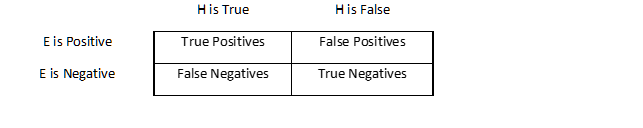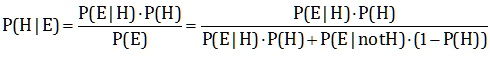Why do people fall prey to the Inverse Fallacy?

This is what I think happens. Empirical knowledge consists in the accumulation of evidence in order to evaluate hypotheses. Any sign that can be related to a hypothesis is a form of evidence. We continuously revise the probability of hypotheses in the light of new evidence.

In general, a hypothesis H can either be true or false. The probability that H is true is P(H) and the probability that it is false is 1-P(H). P(H) is the Base Rate (BR), i.e. the Prior Probability of H before the arrival of new evidence E. When E arrives, it can either be positive (i.e. consistent with H being true) or negative (i.e. consistent with H being false). There are four possible cases:Through direct observation or by other means, we form beliefs about the probabilities of the four cases:

True Positive Rate (TPR) is the probability of observing E if H is true: P(E|H)

False Positive Rate (FPR) is the probability of observing E if H is false: P(E|not H)

True Negative Rate (TNR) is the probability of not observing E if H is false: P(not E|not H)

False Negative Rate (FNR) is the probability of not observing E when H is true: P(not E|H)

Notice that, since E is either positive or negative, TPR=1-FNR and TNR=1-FPR.

Starting with any value of P(H), Bayes’ Theorem tells us how to revise it in the light of new evidence:or, using our alternative notation, the Posterior Probability (PP) of H in the light of E is:The Inverse Fallacy consists in confusing P(H|E) with P(E|H), or PP with TPR. Notice that this happens if BR=50%. In that case:PP is close to TPR since TPR+FPR is typically close or equal to 1.

So the Inverse Fallacy is ultimately a Prior Indifference Fallacy.

In our child football star example, the reasoning was: I don’t really know whether my child is good or not. But he has taken this test, which the coach says is very accurate: in fact it is apparently infallible at spotting top players (TPR=100%), and only rarely mistakes a normal child for a top player (FPR=5%). If the test is really this accurate, my child will very likely have a bright future as a football star: PP ≈TPR.

In other words: Since the test is 100% accurate at spotting future top players, and the test is saying that my child will be a top player, then he will almost surely be one. We saw this was a massively mistaken conclusion: the actual probability was less than 2%. The key to the blunder is in the first sentence: I don’t really know whether my child is good or not. Innocuous as it seems, this is equivalent to Prior Indifference: BR=50%. We are implicitly assuming that, before the test, the child has a 50% chance to turn into a football champion! Such a blatant mistake is strictly dependent on the presence of a test. Without the test, the father would have immediately asked the coach: what is the chance that a child like my son will become a top player? The coach would have given him a small Base Rate, like one in a thousand (0.1%). But as soon as he mentions the test, the father ignores the Base Rate and concentrates his attention entirely on the test result.

Here is the amazing paradox. Without the test, the father would have reckoned his child had a 0.1% probability of becoming a champion. With the test, after a positive response, that probability increased appreciably: it went from 0.1% to 1.96%. But he thought it had gone all the way to 100% or thereabouts. The father used the test because he wanted more evidence to lead him closer to the truth, but he ended up drifting far away from it. He would have stayed much closer to the truth if his child had not taken the test: he was blinded by evidence.

### One Response to “Blinded by evidence”

1.Have not seen this paradox pointed out before – interesting !

This site uses Akismet to reduce spam. Learn how your comment data is processed.